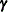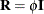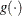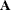#### G-Side and R-Side Random Effects and Covariance Structures

The GLIMMIX procedure distinguishes two types of random effects. Depending on whether the parameters of the covariance structure for random components in your model are contained inor in, the procedure distinguishes between G-side and R-side random effects. The associated covariance structures ofandare similarly termed the G-side and R-side covariance structure, respectively. R-side effects are also called residual effects. Simply put, if a random effect is an element of, it is a G-side effect and you are modeling the G-side covariance structure; otherwise, you are modeling the R-side covariance structure of the model. Models without G-side effects are also known as marginal (or population-averaged) models. Models fit with the GLIMMIX procedure can have none, one, or more of each type of effect.

Note that an R-side effect in the GLIMMIX procedure is equivalent to a REPEATED effect in the MIXED procedure. The R-side covariance structure in the GLIMMIX procedure is the covariance structure that you would formulate with the REPEATED statement in the MIXED procedure. In the GLIMMIX procedure all random effects and their covariance structures are specified through the RANDOM statement. See the section Comparing the GLIMMIX and MIXED Procedures for a comparison of the GLIMMIX and MIXED procedures.

The columns ofare constructed from effects listed on the right side in the MODEL statement. Columns ofand the variance matricesandare constructed from the RANDOM statement.

Thematrix is by default the scaled identity matrix,. The scale parameteris set to one if the distribution does not have a scale parameter, such as in the case of the binary, binomial, Poisson, and exponential distribution (see Table 41.20). To specify a differentmatrix, use the RANDOM statement with the _RESIDUAL_ keyword or the RESIDUAL option. For example, to specify that the Time effect for each patient is an R-side effect with a first-order autoregressive covariance structure, use the RESIDUAL option:

random time / type=ar(1) subject=patient residual;


To add a multiplicative overdispersion parameter, use the _RESIDUAL_ keyword:

random _residual_;


You specify the link functionwith the LINK= option in the MODEL statement or with programming statements. You specify the variance function that controls the matrixwith the DIST= option in the MODEL statement or with programming statements.

Unknown quantities subject to estimation are the fixed-effects parameter vectorand the covariance parameter vectorthat comprises all unknowns inand. The random effectsare not parameters of the model in the sense that they are not estimated. The vectoris a vector of random variables. The solutions forare predictors of these random variables.